2019-09-11 15:04:46 LEILEI18A 阅读数 58
• ###### 玩转深度学习实战教程

玩转深度学习视频培训课程，详细讲解深度学习的原理和利用深度学习框架TensorFlow进行项目实战。课程通过Kaggle竞赛平台的Titanic问题讲解TensorFlow的基本用法以及问题处理的常用技巧，讲解深度学习图像领域的卷积神经网络CNN和多个经典的网络架构、CNN的应用，讲解自然语言处理领域的RNN、LSTM以及它们的多种变种结构，构建语言模型和对话机器人，介绍损失函数和优化深度学习算法在TensorFlow中的实现。

3188 人正在学习 去看看 王而川

## 如何改进和优化深度学习方法？

1. 学术上

2. 工程上

在训练集训练，再和训练集一起划分的测试集上测试，效果很好，但是实际测试效果很差，需要从多方面进行分析，然后才能改进。

从 数据集情况/数据预处理方式/网络结构/损失函数/后处理等方面进行分析。

判断是否为数据集的问题，无论是与不是，都要进一步分析数据预处理方式，要怎么预处理增加一些“情况”，增加亮度等。

2018-03-15 17:24:29 u013498583 阅读数 286
• ###### 玩转深度学习实战教程

玩转深度学习视频培训课程，详细讲解深度学习的原理和利用深度学习框架TensorFlow进行项目实战。课程通过Kaggle竞赛平台的Titanic问题讲解TensorFlow的基本用法以及问题处理的常用技巧，讲解深度学习图像领域的卷积神经网络CNN和多个经典的网络架构、CNN的应用，讲解自然语言处理领域的RNN、LSTM以及它们的多种变种结构，构建语言模型和对话机器人，介绍损失函数和优化深度学习算法在TensorFlow中的实现。

3188 人正在学习 去看看 王而川

SGD

SGD就是每一次迭代计算mini-batch的梯度，然后对参数进行更新，是最常见的优化方法了。即：

${g}_{t}={\mathrm{\nabla }}_{{\theta }_{t-1}}f\left({\theta }_{t-1}\right)$

$\mathrm{\Delta }{\theta }_{t}=-\eta \ast {g}_{t}$

SGD完全依赖于当前batch的梯度，所以\eta可理解为允许当前batch的梯度多大程度影响参数更新

- 对所有的参数更新使用同样的learning rate。对于稀疏数据或者特征，有时我们可能想更新快一些对于不经常出现的特征，对于常出现的特征更新慢一些，这时候SGD就不太能满足要求了

SGD容易收敛到局部最优，并且在某些情况下可能被困在鞍点【原来写的是“容易困于鞍点”，经查阅论文发现，其实在合适的初始化和step size的情况下，鞍点的影响并没这么大。感谢@冰橙的指正】

Momentum
momentum是模拟物理里动量的概念，积累之前的动量来替代真正的梯度。公式如下：

${m}_{t}=\mu \ast {m}_{t-1}+{g}_{t}$

$\mathrm{\Delta }{\theta }_{t}=-\eta \ast {m}_{t}$

Nesterov
nesterov项在梯度更新时做一个校正，避免前进太快，同时提高灵敏度。

$\mathrm{\Delta }{\theta }_{t}=-\eta \ast \mu \ast {m}_{t-1}-\eta \ast {g}_{t}$

${g}_{t}={\mathrm{\nabla }}_{{\theta }_{t-1}}f\left({\theta }_{t-1}-\eta \ast \mu \ast {m}_{t-1}\right)$

${m}_{t}=\mu \ast {m}_{t-1}+{g}_{t}$

$\mathrm{\Delta }{\theta }_{t}=-\eta \ast {m}_{t}$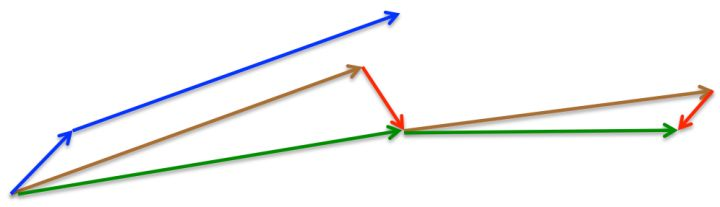momentum首先计算一个梯度(短的蓝色向量)，然后在加速更新梯度的方向进行一个大的跳跃(长的蓝色向量)，nesterov项首先在之前加速的梯度方向进行一个大的跳跃(棕色向量)，计算梯度然后进行校正(绿色梯向量)

${n}_{t}={n}_{t-1}+{g}_{t}^{2}$

$\mathrm{\Delta }{\theta }_{t}=-\frac{\eta }{\sqrt{{n}_{t}+ϵ}}\ast {g}_{t}$

$\eta$$\eta$设置过大的话，会使$regularizer$$regularizer$过于敏感，对梯度的调节太大

${n}_{t}=\nu \ast {n}_{t-1}+\left(1-\nu \right)\ast {g}_{t}^{2}$

$\mathrm{\Delta }{\theta }_{t}=-\frac{\eta }{\sqrt{{n}_{t}+ϵ}}\ast {g}_{t}$

$E|{g}^{2}{|}_{t}=\rho \ast E|{g}^{2}{|}_{t-1}+\left(1-\rho \right)\ast {g}_{t}^{2}$

$\mathrm{\Delta }{x}_{t}=-\frac{\sqrt{\sum _{r=1}^{t-1}\mathrm{\Delta }{x}_{r}}}{\sqrt{E|{g}^{2}{|}_{t}+ϵ}}$

RMSprop

$\rho =0.5$$\rho=0.5$时，$E|{g}^{2}{|}_{t}=\rho \ast E|{g}^{2}{|}_{t-1}+\left(1-\rho \right)\ast {g}_{t}^{2}$$E|g^2|_t=\rho*E|g^2|_{t-1}+(1-\rho)*g_t^2$就变为了求梯度平方和的平均数。

$RMS|g{|}_{t}=\sqrt{E|{g}^{2}{|}_{t}+ϵ}$$RMS|g|_t=\sqrt{E|g^2|_t+\epsilon}$

$\mathrm{\Delta }{x}_{t}=-\frac{\eta }{RMS|g{|}_{t}}\ast {g}_{t}$$\Delta{x_t}=-\frac{\eta}{RMS|g|_t}*g_t$

- 对于RNN效果很好

${m}_{t}=\mu \ast {m}_{t-1}+\left(1-\mu \right)\ast {g}_{t}$

${n}_{t}=\nu \ast {n}_{t-1}+\left(1-\nu \right)\ast {g}_{t}^{2}$

$\stackrel{^}{{m}_{t}}=\frac{{m}_{t}}{1-{\mu }^{t}}$

$\stackrel{^}{{n}_{t}}=\frac{{n}_{t}}{1-{\nu }^{t}}$

$\mathrm{\Delta }{\theta }_{t}=-\frac{\stackrel{^}{{m}_{t}}}{\sqrt{\stackrel{^}{{n}_{t}}}+ϵ}\ast \eta$

- 适用于大数据集和高维空间

${n}_{t}=max\left(\nu \ast {n}_{t-1},|{g}_{t}|\right)$

$\mathrm{\Delta }x=-\frac{\stackrel{^}{{m}_{t}}}{{n}_{t}+ϵ}\ast \eta$

$\stackrel{^}{{g}_{t}}=\frac{{g}_{t}}{1-{\mathrm{\Pi }}_{i=1}^{t}{\mu }_{i}}$

${m}_{t}={\mu }_{t}\ast {m}_{t-1}+\left(1-{\mu }_{t}\right)\ast {g}_{t}$

$\stackrel{^}{{m}_{t}}=\frac{{m}_{t}}{1-{\mathrm{\Pi }}_{i=1}^{t+1}{\mu }_{i}}$

${n}_{t}=\nu \ast {n}_{t-1}+\left(1-\nu \right)\ast {g}_{t}^{2}$

$\stackrel{^}{{n}_{t}}=\frac{{n}_{t}}{1-{\nu }^{t}}\overline{{m}_{t}}=\left(1-{\mu }_{t}\right)\ast \stackrel{^}{{g}_{t}}+{\mu }_{t+1}\ast \stackrel{^}{{m}_{t}}$

$\mathrm{\Delta }{\theta }_{t}=-\eta \ast \frac{\overline{{m}_{t}}}{\sqrt{\stackrel{^}{{n}_{t}}}+ϵ}$

SGD通常训练时间更长，但是在好的初始化和学习率调度方案的情况下，结果更可靠

2017-01-03 12:51:43 yas12345678 阅读数 2338
• ###### 玩转深度学习实战教程

玩转深度学习视频培训课程，详细讲解深度学习的原理和利用深度学习框架TensorFlow进行项目实战。课程通过Kaggle竞赛平台的Titanic问题讲解TensorFlow的基本用法以及问题处理的常用技巧，讲解深度学习图像领域的卷积神经网络CNN和多个经典的网络架构、CNN的应用，讲解自然语言处理领域的RNN、LSTM以及它们的多种变种结构，构建语言模型和对话机器人，介绍损失函数和优化深度学习算法在TensorFlow中的实现。

3188 人正在学习 去看看 王而川

# BGD

1. 提取训练集中的所有内容{x1,,xn},以及相关的输出yi
2. 计算梯度和误差并更新参数:

g^+1nθiL(f(xi;θ),yi)θθϵg^

# SGD

1. 从训练集中的随机抽取一批容量为m的样本{x1,,xm},以及相关的输出yi
2. 计算梯度和误差并更新参数:

g^+1mθiL(f(xi;θ),yi)θθϵg^

k=1ϵk=k=1ϵ2k<

ϵk=(1α)ϵ0+αϵτα=kτ

# Momentum

1. 从训练集中的随机抽取一批容量为m的样本{x1,,xm},以及相关的输出yi
2. 计算梯度和误差,并更新速度v和参数θ

g^+1mθiL(f(xi;θ),yi)vαvϵg^θθ+v

ϵg1α

# Nesterov Momentum

1. 从训练集中的随机抽取一批容量为m的样本{x1,,xm},以及相关的输出yi
2. 计算梯度和误差,并更新速度v和参数θ

g^+1mθiL(f(xi;θ+αv),yi)vαvϵg^θθ+v

ϵn=ϵδ+n1i=1gigi

1. 从训练集中的随机抽取一批容量为m的样本{x1,,xm},以及相关的输出yi
2. 计算梯度和误差,更新r,再根据r和梯度计算参数更新量

g^+1mθiL(f(xi;θ),yi)rr+g^g^θ=ϵδ+rg^θθ+θ

# RMSProp

RMSProp通过引入一个衰减系数，让r每回合都衰减一定比例，类似于Momentum中的做法。

1. 从训练集中的随机抽取一批容量为m的样本{x1,,xm},以及相关的输出yi
2. 计算梯度和误差,更新r,再根据r和梯度计算参数更新量

g^+1mθiL(f(xi;θ),yi)rρr+(1ρ)g^g^θ=ϵδ+rg^θθ+θ

# RMSProp with Nesterov Momentum

1. 从训练集中的随机抽取一批容量为m的样本{x1,,xm},以及相关的输出yi
2. 计算梯度和误差,更新r,再根据r和梯度计算参数更新量

θ~θ+αvg^+1mθ~iL(f(xi;θ~),yi)rρr+(1ρ)g^g^vαvϵrg^θθ+v

1. 从训练集中的随机抽取一批容量为m的样本{x1,,xm},以及相关的输出yi
2. 计算梯度和误差,更新r和s,再根据r和s以及梯度计算参数更新量

g+1mθiL(f(xi;θ),yi)sρ1s+(1ρ1)grρ2r+(1ρ2)ggs^s1ρ1r^r1ρ2θ=ϵs^r^+δθθ+θ

2020-02-23 13:16:33 l_yiyu 阅读数 263
• ###### 玩转深度学习实战教程

玩转深度学习视频培训课程，详细讲解深度学习的原理和利用深度学习框架TensorFlow进行项目实战。课程通过Kaggle竞赛平台的Titanic问题讲解TensorFlow的基本用法以及问题处理的常用技巧，讲解深度学习图像领域的卷积神经网络CNN和多个经典的网络架构、CNN的应用，讲解自然语言处理领域的RNN、LSTM以及它们的多种变种结构，构建语言模型和对话机器人，介绍损失函数和优化深度学习算法在TensorFlow中的实现。

3188 人正在学习 去看看 王而川

# 优化与深度学习

## 1.优化与估计

• 优化方法目标：训练集损失函数值
• 深度学习目标：测试集损失函数值（泛化性）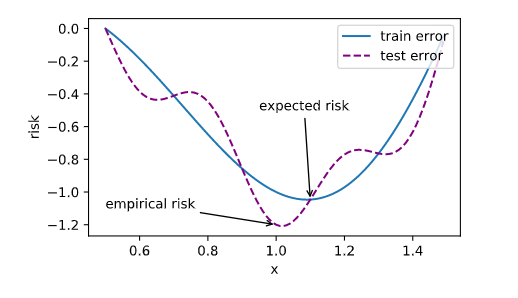## 2.优化在深度学习中的挑战

1. 局部最小值
2. 鞍点
3. 梯度消失

### 2.1局部最小值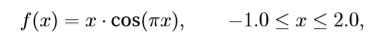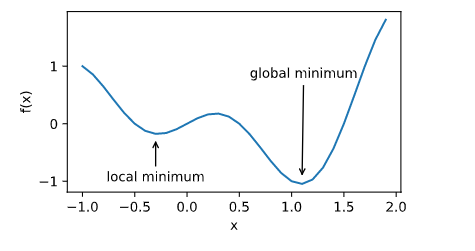### 2.2 鞍点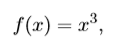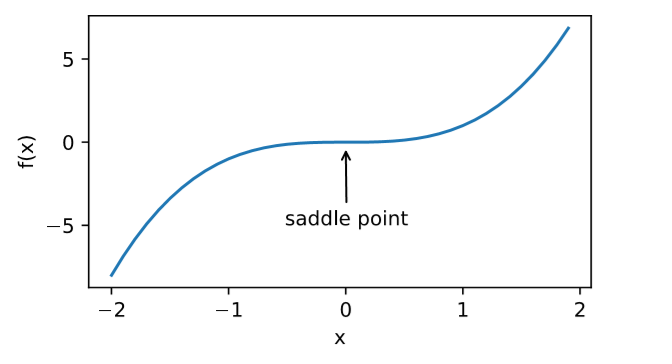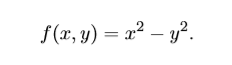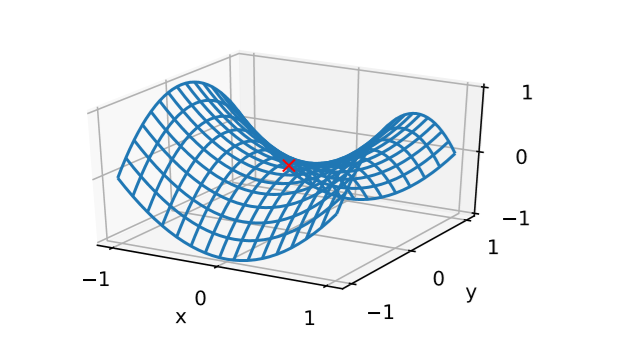• 当函数的⿊塞矩阵在梯度为零的位置上的特征值全为正时，该函数得到局部最小值。

• 当函数的⿊塞矩阵在梯度为零的位置上的特征值全为负时，该函数得到局部最⼤值。

• 当函数的⿊塞矩阵在梯度为零的位置上的特征值有正有负时，该函数得到鞍点。

## 小结：

• 由于优化算法的⽬标函数通常是⼀个基于训练数据集的损失函数，优化的⽬标在于降低训 练误差。

• 由于深度学习模型参数通常都是⾼维的，⽬标函数的鞍点通常⽐局部最小值更常⻅。

2019-07-23 22:07:22 weixin_39853245 阅读数 76
• ###### 玩转深度学习实战教程

玩转深度学习视频培训课程，详细讲解深度学习的原理和利用深度学习框架TensorFlow进行项目实战。课程通过Kaggle竞赛平台的Titanic问题讲解TensorFlow的基本用法以及问题处理的常用技巧，讲解深度学习图像领域的卷积神经网络CNN和多个经典的网络架构、CNN的应用，讲解自然语言处理领域的RNN、LSTM以及它们的多种变种结构，构建语言模型和对话机器人，介绍损失函数和优化深度学习算法在TensorFlow中的实现。

3188 人正在学习 去看看 王而川

## 随机梯度下降SGD: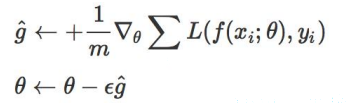• 选择合适的learning rate比较困难：如果数据是稀疏的，我们会想对出现频率低的特征进行快一点的更新，而高频的进行慢一点的更新，这时候SGD就不太能满足要求了
• SGD容易收敛到局部最优，并且极容易被困在鞍点上，在这种点所有反向上的梯度值都为0（如果设置合适的初始化和step size，鞍点的影响会减少很多）

## Momentum:

Momentum是模拟物理里动量的概念，即，前几次的梯度也会参与到运算中，因此：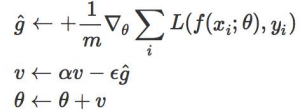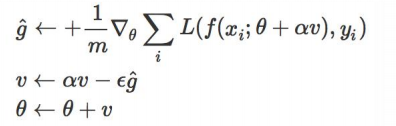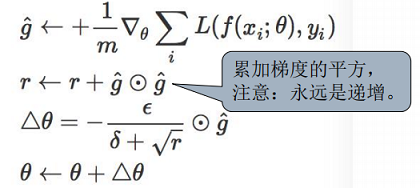## RMSProp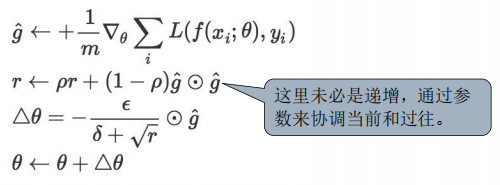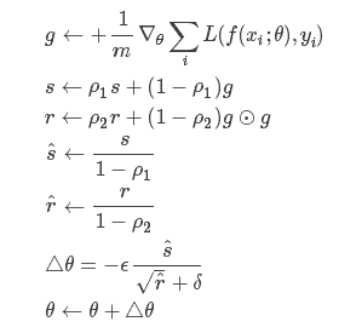## 各种梯度下降算法的比较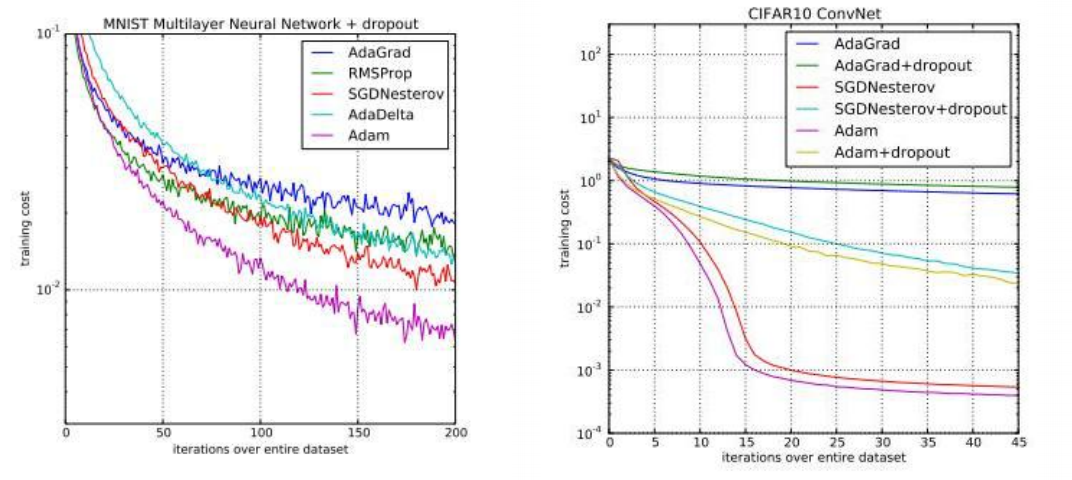• 论文中通常采用不用momentum的SGD。SGD通常训练时间更长，容易陷入鞍点，但是在好的初始化和学习率调度方案的情况下，结果更可靠。
• 在国际顶尖期刊的优化方法中：SGD和momentum的更新方法常用于CNN之类的网络结构；而自适应学习率算法的优化方法常用于RNN之类的网络结构。